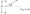# VTU Previous Exam Paper BE EC 6th Sem Antenna and Propagation Feb 06

Note: /. Answer any FIVE full questions.

2.All questions carry equal marks.

3.Any missing data can be suitably assumed.

1.(a) Define aperture. Show that maximum effective aperture of a A/2 dipole is 0.13A2.Also find the directivity.

(b) Derive Friis transmission formula. >

(c) Prove that directivity for a source with a unidirectional power pattern given by U = Ujncosn0 can be expressed as Dn — 2(n -f 1)* U has a value for 0< 9 < 90°. The patterns are independent of azimuth angle.

2.  (a) Find the relative E((j)) pattern of an array of two identical isotropic in phase point sources arranged as shown in figure. Also find the expressions for maxima, nulls and half power points.

(b) For a Broadside array of n isotropic point sources of equal amplitude and spacing, show that \$q = arc cos                                               where (j>0 gives the null directions. Find null directions for an array of 4 isotropic point sources with A/2 spacing.

(c) State and explain the principle otpattern multiplication. Calculate and plot the field pattern of an array of two nonisotropic dissimilar sources for which the totai field is given by E — cos(j> + sin<j> j tJ) where •</> = dcostj) -j- S — y(cos<\$ -j-1)

Take source 1 as reference as shown in figure.Q2.Cc)

3. (a) Starting from the fundamentals derive the expressions for the far field components of thin linear antenna.

(b) A dipole antenna of length 5cm is operated at a frequency of 100MHz with a terminal current /0 = 120mA a\t — 1 sec, 6 — 45° and distance r = 3m,

Find i) i) Eq ii) H(j}

(c) Using Poynting vector integration, show that the radiation resistance of a   smai! loop’ iis equal to 3207T4 f j il,

4. (a) Describe a helical antenna : Discuss the properties of. two modes of operation.

Explain how linearly polarised radiation may be obtained using helical antenna.

(b) Design a Yagi-Uda six element antenna for operation at 500MHz with a folded dipole feed. What are the lengths of

i) reflector element

ii) driven element

iii) four director elements ? What is spacing

iv) between reflector and driven element and

v)between director elements ?

(c) How is slot antenna excited ? Give some typical applications of slot antenna.

5. (a) Show that the field intensity ratio in the aperture plane of a cylindrical parabolic reflector is \ f —±-4

(b)Explain in detail the log periodic antenna What are their advantages?

(c) What is the approximate directivity of a rectangular horn antenna, whose physical aperture is 81 A2?

6. (a) Show that radius of curvature of path is a function of the rate of change of dielectric constant with height.

(b) Find the approximate formula for the field strength in VHF propagation.

(c) Two aircrafts are flying at altitudes of 3km and 6km respectively. What is the maximum possible distance aiong the surface of the earth overwhich they can have effective point to point communication?

7. (a) Obtain the expression for refractive index of the ionosphere in the case of ionosphericpropagation.

(b) Define maximum usable frequency (MUF). Find an expression to calculate MUF.

(c)  What is meant by critical frequency of ionospheric layer ? Develop an expression for critical frequency. Calculate electron density required to a return signal at 12MHz incident on the bottom of the layer at an angle 26° to the normal.

8. Write short notes on :

a)  Babinet’s principle

b)  Skip distance

c)   Embedded antenna

d)   Plasma antenna